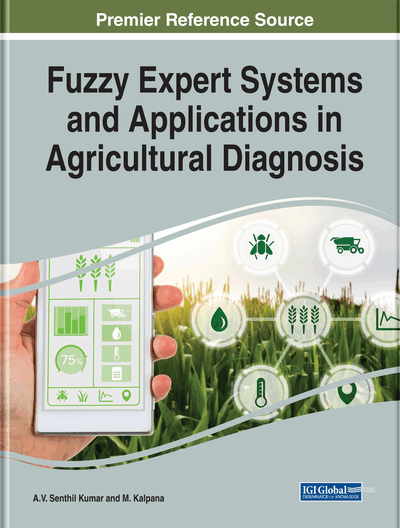# Adaptive Neuro-Fuzzy Inference System in Agriculture

K. Aditya Shastry, Sanjay H. A.
DOI: 10.4018/978-1-5225-9175-7.ch008
OnDemand:
(Individual Chapters)
Available
\$29.50
No Current Special Offers

## Abstract

This chapter emphasizes the use of adaptive fuzzy inference system (ANFIS) in agriculture. An overview of the basic concepts of ANFIS is provided at the beginning, where the underlying architecture of ANFIS is also discussed. The introduction is followed by the second section which highlights the diverse applications of ANFIS in agriculture during recent times. The third section describes how Matlab software can be utilized to build the ANFIS model. The fourth section describes the case study of the application of ANFIS for crop yield prediction. The conclusion follows this case study.
Chapter Preview
Top

## Introduction

ANFIS represents a category of the artificial neural network (ANN) which was founded during the 1990s (Jyh-Shing and R. Jang, 1991). It can capture the strengths of ANN and principles of fuzzy logic into a single framework. The ANFIS possesses an inference system comprising of many fuzzy IF-THEN rules which can learn nonlinear functions (Jyh-Shing and Roger Jang, 1993) due to which ANFIS is called as a universal estimator. The genetic algorithm can be utilized to choose the optimal parameters of the ANFIS (Jyh-Shing et al., 1997).

Both fuzzy logic (FL) and neural networks are universal estimators as they can be utilized for function estimation up to some predefined accuracy, provided an adequate number of fuzzy rules and hidden neurons are available. In ANFIS, the gradient descent (GD) and back-propagation (BP) algorithms are utilized to tune the ANFIS parameters such as the membership functions (MF) and the neural network weights (defuzzification). Fuzzification and defuzzification are the two methods for updating the ANFIS parameters. The efficient integration of significant features of FL and ANN is the primary objective of ANFIS. The benefit of FL is that prior knowledge is signified as a set of constraints. Furthermore, the accuracy of ANFIS on test data may be improved if the number of its adjustable parameters is at least equal to the size of the training data (Shodhganga, 2018).

The two soft computing methodologies namely, fuzzy logic (FL) and ANN are combined to form ANFIS. FL can map the qualitative features of human knowledge and perceptions into accurate, quantifiable components. Nevertheless, it does not have a specific technique which could be utilized as a guide for transforming human knowledge into a rule-based fuzzy inference system (FIS). The tuning of membership functions (MFs) takes much time. However, it possesses a better capability to adjust to diverse environments during its learning process than ANN.

Consequently, the ANN could be utilized to automatically fine-tune the MFs and decrease the error rates for creating the fuzzy logic rules. Three critical components of FIS are the rule base that comprises of choosing fuzzy logic rules of the form “If-Then,” a database that expresses the MFs to be used in fuzzy rules and reasoning mechanisms to provide the final inference. ANFIS uses hybrid training algorithm which is a grouping of back-propagation and least mean square methods. Its primary purpose is to reduce the error due to approximation. When FIS is trained, many iterations are employed which reduce the error. As the number of iteration increases, the error starts to decrease. In ANFIS, iterations are utilized to tune the weights for decreasing the errors (Suparta & Alhasa, 2016). The chief advantages of ANFIS are highlighted below:

• To define the behavior of a complex system – Complex systems such as crop yield prediction in agriculture, soil classification, the circuit design can be modeled adequately by ANFIS.

• Improvement of fuzzy if-then rules – The FIS can be improved using the ANFIS model as it adds membership functions to the rules.

• No need for prior human expertise – Human knowledge is not required for the initial development of the ANFIS model

• Greater choice of MFs to use – Different types of MFs like a trapezoid, triangular are available which offer diverse functions.

• Fast convergence time- The converging time of ANFIS to obtain the final solution is observed to be faster than other models.

Top

## Background

The previous works for predicting the yields of various crops like rice, wheat using different prediction models are surveyed in this section. Table 1 reviews the recent developments done in the agricultural domain using ANFIS.

## Key Terms in this Chapter

Fuzzy Set: Fuzzy set is expressed as a function and the elements of the set are mapped into their degree of membership. A set with the fuzzy boundaries are “hot,” “medium,” or “cold” for temperature.

Artificial Neural Network (ANN): ANN represents a set of algorithms which are designed for pattern recognition. It is modelled after the human brain.

Degree of Membership: It represents a value in the range of 0 and 1. A value closer to 1 indicates that an element belongs to a particular class, while values closer to 0 indicate that the element doesn’t belong to the particular class.

Antecedent: Antecedent is a part of IF rule. It is a conditional statement.

Fuzzy Inference System (FIS): The FIS is used for mapping the inputs to outputs by utilizing the fuzzy set theory. The inputs and outputs represent features and classes respectively with regards to fuzzy classification.

Membership Function (MF): MF signifies the degree to which each input feature is mapped to a membership value between 0 and 1. It is basically a curve.

Fuzzy Logic (FL): It is a multi-valued logic which deals with the concept of partial truth. It is used in cases where the conditions cannot be described by exact values of 0 (false) and 1 (true). The values can lie between 0 and 1.

## Complete Chapter List

Search this Book:
Reset